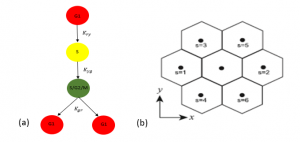Understanding the parameters which govern the cell cycle and spread of cells has wide impacts in many areas. One being cancer research, where drug treatments can be more effective during different phases of the cell cycle (Haass & Gabrielli, 2017). This project develops a parameter estimation method for a stochastic model of melanoma skin cells utilising fluorescent ubiquitination-based cell cycle indicator (FUCCI) technology to visualise the cell cycle. Investigations are based on simulated data.

Firstly, the cell cycle for melanoma skin cells is conceptualised as a sequence of four stages: gap 1 (G1); synthesis (S); gap 2 (G2); and the mitotic (M) phase (Haass, 2014). During the mitotic phase the cell will divide into two daughter cells and begin the cell cycle again. FUCCI technology is able to visualise the cell cycle into three of these phases; by emitting a red fluorescent when the cells are in the G1 phase, a green fluorescent when the cells are in S/G2/M phase and appear yellow when in early S phase (see Figure 1 (a)). Using the Gillespie algorithm, the simulation model (developed by Simpson et al) simulates a 2D hexagonal lattice random walk (see Figure 1 (b)) which is dependent on the cell proliferation rates and motility rates. In this project I estimate the posterior distribution for the proliferation rates using Approximate Bayesian Computation (ABC) methods. I rely on this method because the likelihood function for the melanoma skin cells is intractable (often the case with stochastic random walk models) which makes conventional Bayesian inference methods infeasible.Figure 1: (a) Schematic showing the progression of cell cycle for FUCCI. (b) Hexagonal Lattice (Simpson et al, 2018)

ABC involves summarising observed data and simulated data and comparing their difference by some appropriate discrepancy function. Taking the count of each cell subpopulation (red, yellow, green) as summary statistics I compared observed and simulated data using the Euclidean distance as the discrepancy function. However, in place of using observed data, I simulated a sample of data derived from arbitrary parameter values which we assume to be true and then took the average of the samples as our “observed” data. This way we know the true values which the marginal posterior distributions should be centred around. There are a few algorithm choices which I explored to attain the posterior distribution but the Sequential Monte Carlo Approximate Bayesian Computation (SMC ABC) replenishment algorithm (Drovandi & Pettitt, 2011) was preferable due to its greater efficiency over other algorithms such as Monte Carlo Markov Chain ABC (MCMC ABC) (Marjoram, 2003; Sisson & Fan, 2011).

Using the SMC ABC algorithm and the summary statistics recorded at 48 hours, I was able to sample from the posterior distribution and produce marginal posterior distributions which contain the parameter values we assumed to be true. I was able to increase the precision of the algorithm by including a second time point at 24 hours for the summary statistics. Finally, I found that using the count of the cell subpopulations as a summary statistic was reliable independently to experimental design by repeating the algorithm for multiple initial seeds.

References

         Drovandi, C & Pettitt, A 2011, ‘Estimation of Parameters for Macroparasite Population Evolution Using Approximate Bayesian Computation’, Biometrics, vol. 67, no. 1, pp. 225-233

         Gillespie, D 1977, ‘Exact stochastic simulation of coupled chemical reaction, The Journal of Physical Chemistry’, vol. 81, no. 25, pp. 2340-2361

         Haass, N & Gabrielli, B 2017, ‘Cell cycle- tailored targeting of metastatic melanoma: Challenges and opportunities’, Experimental Dermatology, vol. 365, pp. 189-195

         Haass, N et al 2014, ‘Real‐time cell cycle imaging during melanoma growth, invasion, and drug response’, Pigment Cell & Melanoma Research, vol. 27, no. 1, pp. 764–776.

         Marjoram, P et al. 2003, ‘Markov chain Monte Carlo without likelihoods’, Proceedings of the National Academy of Sciences of the United States of America, vol. 100, no. 26, pp. 15324– 15328

         Simpson, M et al 2018, ‘Stochastic models of cell invasion with fluorescent cell cycle Indicators’, Physica A, vol. 210, pp. 375-386

         Sisson, S. A. & Fan, Y. 2011, ‘Likelihood-free MCMC’, in Handbook of Markov Chain Monte Carlo. CRC Press. pp. 313–336

Michael Carr
Queensland University of TechnologyView Michael Carr
Profile